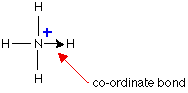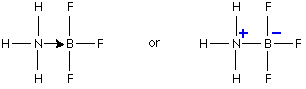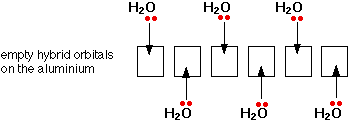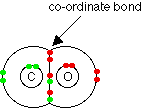# Coordinate (Dative Covalent) Bonding

$$\newcommand{\vecs}{\overset { \rightharpoonup} {\mathbf{#1}} }$$ $$\newcommand{\vecd}{\overset{-\!-\!\rightharpoonup}{\vphantom{a}\smash {#1}}}$$$$\newcommand{\id}{\mathrm{id}}$$ $$\newcommand{\Span}{\mathrm{span}}$$ $$\newcommand{\kernel}{\mathrm{null}\,}$$ $$\newcommand{\range}{\mathrm{range}\,}$$ $$\newcommand{\RealPart}{\mathrm{Re}}$$ $$\newcommand{\ImaginaryPart}{\mathrm{Im}}$$ $$\newcommand{\Argument}{\mathrm{Arg}}$$ $$\newcommand{\norm}{\| #1 \|}$$ $$\newcommand{\inner}{\langle #1, #2 \rangle}$$ $$\newcommand{\Span}{\mathrm{span}}$$ $$\newcommand{\id}{\mathrm{id}}$$ $$\newcommand{\Span}{\mathrm{span}}$$ $$\newcommand{\kernel}{\mathrm{null}\,}$$ $$\newcommand{\range}{\mathrm{range}\,}$$ $$\newcommand{\RealPart}{\mathrm{Re}}$$ $$\newcommand{\ImaginaryPart}{\mathrm{Im}}$$ $$\newcommand{\Argument}{\mathrm{Arg}}$$ $$\newcommand{\norm}{\| #1 \|}$$ $$\newcommand{\inner}{\langle #1, #2 \rangle}$$ $$\newcommand{\Span}{\mathrm{span}}$$$$\newcommand{\AA}{\unicode[.8,0]{x212B}}$$

A coordinate bond (also called a dative covalent bond) is a covalent bond (a shared pair of electrons) in which both electrons come from the same atom. A covalent bond is formed by two atoms sharing a pair of electrons. The atoms are held together because the electron pair is attracted by both of the nuclei. In the formation of a simple covalent bond, each atom supplies one electron to the bond - but that does not have to be the case.

## The Reaction Between Ammonia and Hydrogen Chloride

If these colorless gases are allowed to mix, a thick white smoke of solid ammonium chloride is formed.

The reaction is

$\ce{NH3 (g) + HCl (g) \rightarrow NH4Cl (s)} \nonumber$

Ammonium ions, NH4+, are formed by the transfer of a hydrogen ion (a proton) from the hydrogen chloride molecule to the lone pair of electrons on the ammonia molecule.When the ammonium ion, NH4+, is formed, the fourth hydrogen is attached by a dative covalent bond, because only the hydrogen's nucleus is transferred from the chlorine to the nitrogen. The hydrogen's electron is left behind on the chlorine to form a negative chloride ion. Once the ammonium ion has been formed it is impossible to tell any difference between the dative covalent and the ordinary covalent bonds. Although the electrons are shown differently in the diagram, there is no difference between them in reality.In simple diagrams, a coordinate bond is shown by an arrow. The arrow points from the atom donating the lone pair to the atom accepting it.

##### Example $$\PageIndex{1}$$: Dissolving $$HCl_{(g)}$$ in Water to make Hydrochloric Acid

Something similar happens. A hydrogen ion (H+) is transferred from the chlorine to one of the lone pairs on the oxygen atom.\[ \ce{H_2O + HCl \rightarrow H_3O^{+} + Cl^{-}} \nonumber]

The H3O+ ion is variously called the hydroxonium ion, the hydronium ion or the oxonium ion. In an introductory chemistry course, whenever you have talked about hydrogen ions (for example in acids), you have actually been talking about the hydroxonium ion. A raw hydrogen ion is simply a proton, and is far too reactive to exist on its own in a test tube.

If you write the hydrogen ion as $$\ce{H^{+}(aq)}$$, the "$$\ce{(aq)}$$" represents the water molecule that the hydrogen ion is attached to. When it reacts with something (an alkali, for example), the hydrogen ion simply becomes detached from the water molecule again. Note that once the coordinate bond has been set up, all the hydrogens attached to the oxygen are exactly equivalent. When a hydrogen ion breaks away again, it could be any of the three.

## Reaction between ammonia and boron trifluoride

Boron trifluoride is a compound that does not have a noble gas structure around the boron atom (a notorious "octet violator"). The boron only has three pairs of electrons in its bonding level, whereas there would be room for four pairs. $$BF_3$$ is described as being electron deficient. The lone pair on the nitrogen of an ammonia molecule can be used to overcome that deficiency, and a compound is formed involving a coordinate bond.Using lines to represent the bonds, this could be drawn more simply as:The second diagram shows another way that you might find coordinate bonds drawn. The nitrogen end of the bond has become positive because the electron pair has moved away from the nitrogen towards the boron - which has therefore become negative. We shall not use this method again - it's more confusing than just using an arrow.

## The structure of Aluminum Chloride

Aluminum chloride sublimes (phase transition from solid to gas) at about 180°C. If it simply contained ions it would have a very high melting and boiling point because of the strong attractions between the positive and negative ions. The implication is that it when it sublimes at this relatively low temperature, it must be covalent. The dots-and-crosses diagram shows only the outer electrons.Lewis dot diagram for $$AlCl_3$$

AlCl3, like BF3, is electron deficient. There is likely to be a similarity, because aluminum and boron are in the same group of the Periodic Table, as are fluorine and chlorine.

Measurements of the relative formula mass of aluminum chloride show that its formula in the vapor at the sublimation temperature is not AlCl3, but Al2Cl6. It exists as a dimer (two molecules joined together). The bonding between the two molecules is coordinate, using lone pairs on the chlorine atoms. Each chlorine atom has 3 lone pairs, but only the two important ones are shown in the line diagram.The uninteresting electrons on the chlorines have been faded in color to make the coordinate bonds show up better. There's nothing special about those two particular lone pairs - they just happen to be the ones pointing in the right direction.

Energy is released when the two coordinate bonds are formed, and so the dimer is more energetically stable than two separate AlCl3 molecules.

## The bonding in hydrated metal ions

Water molecules are strongly attracted to ions in solution - the water molecules clustering around the positive or negative ions. In many cases, the attractions are so great that formal bonds are made, and this is true of almost all positive metal ions. Ions with water molecules attached are described as hydrated ions.

Although aluminum chloride is a covalent compound, when it dissolves in water, ions are produced. Six water molecules bond to the aluminum to give an ion with the formula Al(H2O)63+. It's called the hexaaquaaluminum complex ion with as six ("hexa") water molecules ("aqua") wrapped around an aluminum ion. The bonding in this (and the similar ions formed by the great majority of other metals) is coordinate (dative covalent) using lone pairs on the water molecules.

The electron configuration of aluminum is 1s22s22p63s23px1. When it forms an Al3+ ion it loses the 3-level electrons to leave 1s22s22p6. That means that all the 3-level orbitals are now empty. The aluminum reorganizes (hybridizes) six of these (the 3s, three 3p, and two 3d) to produce six new orbitals all with the same energy. These six hybrid orbitals accept lone pairs from six water molecules.

You might wonder why it chooses to use six orbitals rather than four or eight or whatever. Six is the maximum number of water molecules it is possible to fit around an aluminum ion (and most other metal ions). By making the maximum number of bonds, it releases most energy and is the most energetically stable.Only one lone pair is shown on each water molecule. The other lone pair is pointing away from the aluminum and so is not involved in the bonding. The resulting ion looks like this:Because of the movement of electrons towards the center of the ion, the 3+ charge is no longer located entirely on the aluminum, but is now spread over the whole of the ion.

Dotted arrows represent lone pairs coming from water molecules behind the plane of the screen or paper. Wedge shaped arrows represent bonds from water molecules in front of the plane of the screen or paper.

##### Example $$\PageIndex{2}$$: Carbon Monoxide

Carbon monoxide, CO, can be thought of as having two ordinary covalent bonds between the carbon and the oxygen plus a coordinate bond using a lone pair on the oxygen atom.##### Example $$\PageIndex{3}$$: Nitric Acid

In nitric acid, HNO3, one of the oxygen atoms can be thought of as attaching to the nitrogen via a coordinate bond using the lone pair on the nitrogen atom.In fact this structure is misleading because it suggests that the two oxygen atoms on the right-hand side of the diagram are joined to the nitrogen in different ways. Both bonds are actually identical in length and strength, and so the arrangement of the electrons must be identical. There is no way of showing this using a dots-and-crosses picture. The bonding involves delocalization.